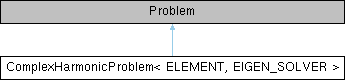ComplexHarmonicProblem< ELEMENT, EIGEN_SOLVER > Class Template Reference

1D ComplexHarmonic problem in unit interval. More...

Inheritance diagram for ComplexHarmonicProblem< ELEMENT, EIGEN_SOLVER >:## Public Member Functions

ComplexHarmonicProblem (const unsigned &n_element)
Constructor: Pass number of elements and pointer to source function. More...

~ComplexHarmonicProblem ()
Destructor. Clean up the mesh and solver. More...

void solve (const unsigned &label)
Solve the problem. More...

void doc_solution (const unsigned &label)
Doc the solution, pass the number of the case considered, so that output files can be distinguished. More...

## Detailed Description

template<class ELEMENT, class EIGEN_SOLVER>
class ComplexHarmonicProblem< ELEMENT, EIGEN_SOLVER >

1D ComplexHarmonic problem in unit interval.

Definition at line 349 of file complex_harmonic.cc.

## ◆ ComplexHarmonicProblem()

template<class ELEMENT , class EIGEN_SOLVER >
 ComplexHarmonicProblem< ELEMENT, EIGEN_SOLVER >::ComplexHarmonicProblem ( const unsigned & n_element )

Constructor: Pass number of elements and pointer to source function.

Constructor for 1D ComplexHarmonic problem in unit interval. Discretise the 1D domain with n_element elements of type ELEMENT. Specify function pointer to source function.

Definition at line 377 of file complex_harmonic.cc.

## ◆ ~ComplexHarmonicProblem()

template<class ELEMENT , class EIGEN_SOLVER >
 ComplexHarmonicProblem< ELEMENT, EIGEN_SOLVER >::~ComplexHarmonicProblem ( )
inline

Destructor. Clean up the mesh and solver.

Definition at line 357 of file complex_harmonic.cc.

## ◆ doc_solution()

template<class ELEMENT , class EIGEN_SOLVER >
 void ComplexHarmonicProblem< ELEMENT, EIGEN_SOLVER >::doc_solution ( const unsigned & label )

Doc the solution, pass the number of the case considered, so that output files can be distinguished.

Doc the solution in tecplot format. Label files with label.

Definition at line 416 of file complex_harmonic.cc.

## ◆ solve()

template<class ELEMENT , class EIGEN_SOLVER >
 void ComplexHarmonicProblem< ELEMENT, EIGEN_SOLVER >::solve ( const unsigned & label )

Solve the problem.

Solve the eigenproblem.

Definition at line 439 of file complex_harmonic.cc.

Referenced by main().

The documentation for this class was generated from the following file: